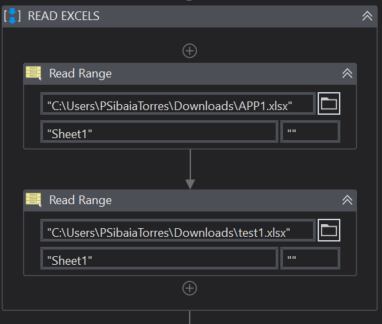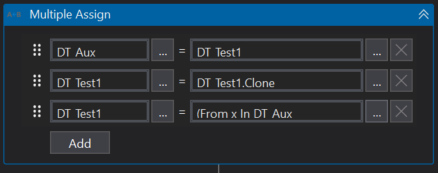# I want to compare two excels and update the data in third sheet in excel where each sheet contains 100k line items

for each in for each with if condition taking too long to process

@Palaniyappan
@Pamela_Sibaja

@Ranganathan_M

Can you give more details on
What you want to compre…is it a row to row match?

Or the rows might be different?

Cheers

I have two excels where i’ll compare three columns data are same . then i will take one column from sheet one
one column from sheet 2

i have used for each row in data table its good for min line items

now i have 1 lakh line items in each sheets… its still processing for last 24 hrs.

kindly give me some solution to process the data in short time.

@Ranganathan_M

Why dont you use excel formula to compare?

You can write a if condition with cells from different sheets using write cell…then use auto fill range to fill the data till the end

Thsi way the processing time reduces a lot…

You can as well try using vlookup formula…if the rows are not matching

Cheers

i wanna update the result data in template which iam creating via BDT

@Ranganathan_M

Yes even then we can still use it…

Once downloaded use copy sheet and copy both sheets to same excel where 3rd sheet is there and then use write cell to write the required formula…and then auto fill range to fill it till end

Cheers

Hi @Ranganathan_M,
You can follow the steps below:

1. Read range the data to extract into data table for the two sheets.2. Use a multiple assign where you can use LINQ Query to compare Datatable1 and Datatable2 where it will be the fastest way to manipulate data3. In the first conditional you can make the comparison according to the Datatable1 and Datatable2 and in the last line you can put the columns of the data table.

CODE:
(From x In DT_Aux
Let comments = If ( DT_APP1.AsEnumerable.Where(Function(y) y.item(“Sr.no”).tostring = x.item(“Sr.no”).tostring).ToArray.COUNT>0,
“Matched”,
“Not Matched”)